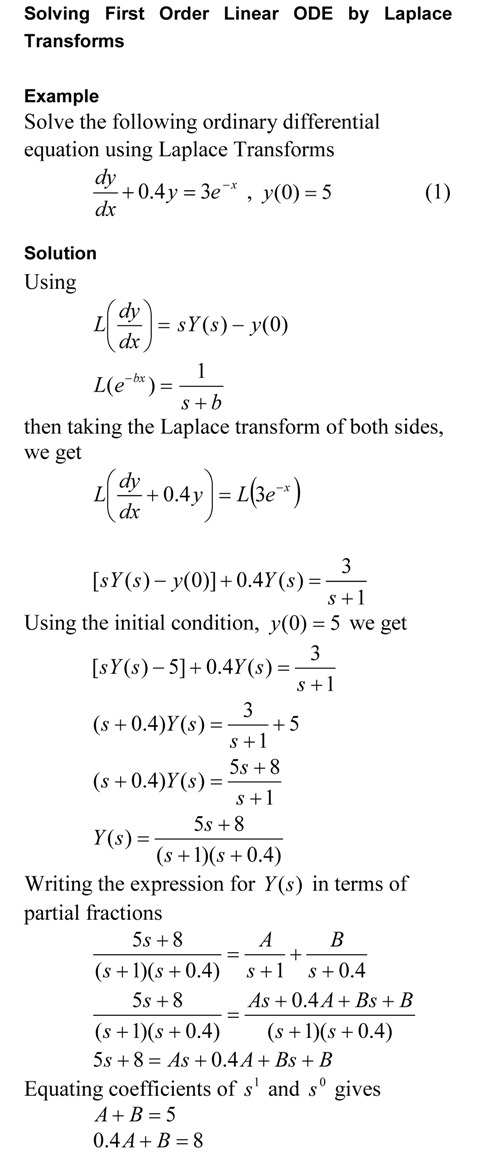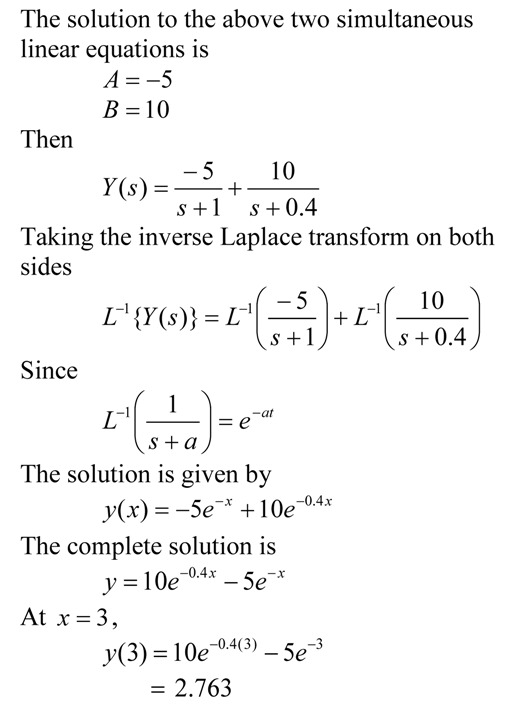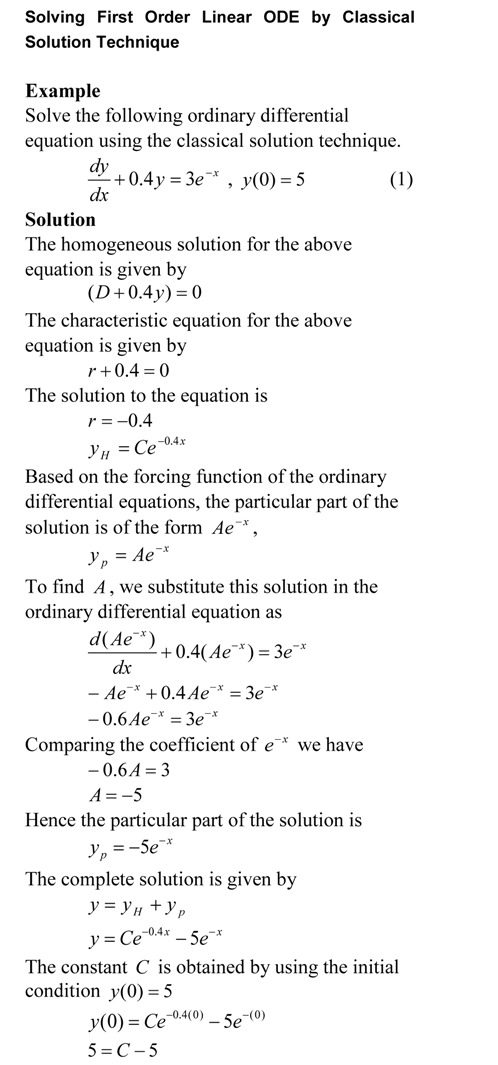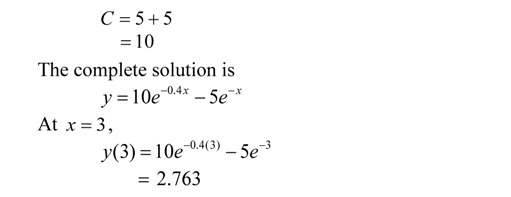## Solution to ordinary differential equations posed as definite integral

This blog is an example to show the use of second fundamental theorem of calculus in posing a definite integral as an ordinary differential equation.  This plays a prominent role in showing how we can use numerical methods of ordinary differential equations to conduct numerical integration.

This post is brought to you by

• Holistic Numerical Methods Open Course Ware:
• the textbooks on
• the Massive Open Online Course (MOOCs) available at

## Reducing ordinary differential equations to state variable matrix form

To be able to solve differential equations numerically, one has to reduce them to a set of first order ordinary differential equations – also called the state variable form.  By writing them in a matrix form, the equations become conducive for programming in languages such as MATLAB.  Here is an example of this reduction to state variable matrix form.This post is brought to you by

• Holistic Numerical Methods Open Course Ware:
• the textbooks on
• the Massive Open Online Course (MOOCs) available at

## An FE Exam Math Problem in Ordinary Differential Equations

“The Fundamentals of Engineering (FE) exam is generally the first step in the process of becoming a professional licensed engineer (P.E.). It is designed for recent graduates and students who are close to finishing an undergraduate engineering degree from an EAC/ABET-accredited program” – FE Exam NCEES

For most engineering majors, mathematics is a required part of the examination. Here is a question from ordinary differential equations.This post is brought to you by

• Holistic Numerical Methods Open Course Ware:
• the textbooks on
• the Massive Open Online Course (MOOCs) available at

## Euler’s Method Example for FE Exam

“The Fundamentals of Engineering (FE) exam is generally the first step in the process of becoming a professional licensed engineer (P.E.). It is designed for recent graduates and students who are close to finishing an undergraduate engineering degree from an EAC/ABET-accredited program” – FE Exam NCEES

For most engineering majors, numerical methods is a required portion of the math part of the examination. Here is an example of using Euler’s method to numerically solve an ordinary differential equation.This post is brought to you by

• Holistic Numerical Methods Open Course Ware:
• the textbooks on
• the Massive Open Online Course (MOOCs) available at

## Global truncation error in Euler’s method

Illustrate through an example that the global truncation error in Euler’s method is proportional to the step size.This post is brought to you by

• Holistic Numerical Methods Open Course Ware:
• the textbooks on
• the Massive Open Online Course (MOOCs) available at

## Local truncation error is approximately proportional to square of step size in Euler’s method

Question: Show that the local truncation error in Euler’s method is proportional to the square of the step size.This post is brought to you by

• Holistic Numerical Methods Open Course Ware:
• the textbooks on
• the Massive Open Online Course (MOOCs) available at

## Repeated roots in ordinary differential equation – next independent solution – where does that come from?

When solving a fixed-constant linear ordinary differential equation where the characteristic equation has repeated roots, why do we get the next independent solution in the form of x^n*e^(m*x)?  Show this through an example.

See this pdf file for the answer.

________________________
This post is brought to you by

## Example: Solving a first order ODE by Laplace transforms

I have a audiovisual digital lecture on YouTube that shows the use of Euler’s method to solve a first order ordinary differential equation (ODE).  To show the accuracy of Euler’s method,  I compare the approximate answer to the exact answer.  A YouTube viewer asked me: How did I get the exact answer?

In this blog, I use the Laplace transform technique to find the exact answer to the ODE.  In a previous blog, I showed how to find the exact answer to the ODE by the classicial solution technique.The pdf file of the solution is also available.

## Classical Solution Technique to Solve a First Order ODE

I have a audiovisual digital lecture on YouTube that shows the use of Euler’s method to solve a first order ordinary differential equation (ODE).  To show the accuracy of Euler’s method,  I compare the approximate answer to the exact answer.  A YouTube viewer asked me: How did I get the exact answer?

In this blog, I use the classical solution technique to find the exact answer to the ODE.  In a previous blog, I showed how to find the exact answer to the ODE by the integrating factor method.The pdf file of the solution is also available.